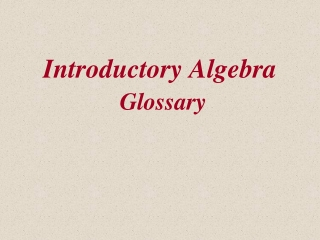DownloadDownload PresentationIntroductory Algebra Glossary

# Introductory Algebra Glossary

Télécharger la présentation## Introductory Algebra Glossary

- - - - - - - - - - - - - - - - - - - - - - - - - - - E N D - - - - - - - - - - - - - - - - - - - - - - - - - - -
##### Presentation Transcript

1. Introductory AlgebraGlossary

2. quadratic equation A quadratic equation is anequation that can be written in the form ax2 + bx + c = 0, where a, b, and c are real numbers,with a 0.

3. discriminant The quantity under the radical in the quadratic formula, b2 - 4ac.

4. complex number Any number that can be written in the form a + bi, where a and b are real numbers.

5. imaginary number A complex number a + bi with b  0.

6. real part The real part of a complex number a + bi is a.

7. imaginary part The imaginary part of the complex number a + bi is b.

8. standard form of a complex number The standard form of a complex number is a + bi.

9. conjugate of a complex number The conjugate of a complex number a + bi is a - bi.

10. parabola The graph of a second-degree (quadratic) equation in two variables is called a parabola.

11. parabola The graph of a second-degree (quadratic) equation in two variables is called a parabola.

12. axis (axis of symmetry) The axis of a parabola is thevertical or horizontal line through the vertex of the parabola.

13. quadratic function A function defined by an equation of the form f(x) = ax2 + bx + c, for real numbers a, b, and c, with a 0.